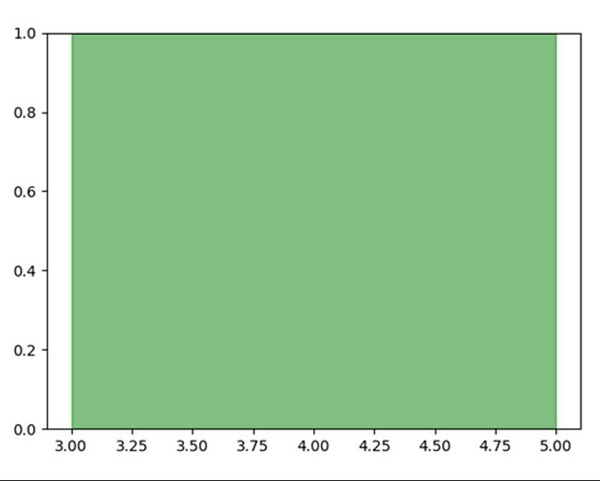# Fill between two vertical lines in matplotlib

To fill color between two vertical lines, use the following steps −

• Using plt.subplots() method, create a figure and a set of subplots. This utility wrapper makes it convenient to create common layouts of subplots, including the enclosing figure object, in a single call.

• To draw two vertical lines, initialize x = 3 and x = 5.

• Using the created ax, axvspan would help to add vertical span(rectangle) across the axes.

This rectangle spans from xmin to xmax horizontally, and, by default, the whole Y-axis vertically.

• To show the figure, use the plt.show() method.

## Example

import matplotlib.pyplot as plt

fig, ax = plt.subplots()

line1 = 3 # vertical x = 3
line2 = 5 # vertical x = 5

ax.axvspan(line1, line2, alpha=.5, color='green')

plt.show()

## Output